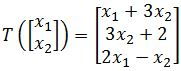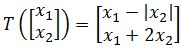# Properties of linear transformation

### Properties of linear transformation

#### Lessons

Recall from last chapter the 2 properties of $Ax$:
1. $A(u+v)=Au+Av$
2. $A(cu)=c(Au)$

where $u$ and $v$ are vectors in $\Bbb{R}^n$ and $c$ is a scalar.

Now the properties of linear transformation are very similar. Linear transformation preserves the operations of vector addition/subtraction and scalar multiplication. In other words, If T is linear, then:
1. $T(u+v)=T(u)+T(v)$
2. $T(cu)=cT(u)$
3. $T(\vec{0})=\vec{0}$

We can even combine property 1 and 2 to show that:

$T(cu+dv)=cT(u)+dT(v)$

where $u$, $v$ are vectors and $c$, $d$ are scalars. Note that if this equation holds, then it must be linear.

If you have more than 2 vectors and 2 scalars? What if you have p vectors and p scalars? Then we can generalize this equation and say that:

$T(c_1 v_1+c_2 v_2+\cdots+c_p v_p )=c_1 T(v_1 )+c_2 T(v_2 )+\cdots+c_p T(v_p)$

Again if this equation holds, then it must be linear.
• Introduction
Properties of Linear Transformation Overview:
a)
The 3 properties of Linear Transformation
$T(u+v)=T(u)+T(v)$
$T(cu)=cT(u)$
$T(0)=0$

b)
How to see if a transformation is linear
• Show that: $T(cu+dv)=cT(u)+dT(v)$
• General formula: $T(c_1 v_1+c_2 v_2+\cdots+c_n v_n )=c_1 T(v_1 )+c_2 T(v_2 )+\cdots+c_p T(v_p)$

• 1.
Understanding and Using the Properties
Show that the transformation $T$ defined byis not linear.

• 2.
Show that the transformation $T$ defined byis not linear.

• 3.
Proving Questions using the Properties
An affine transformation $T: \Bbb{R}^n$$\Bbb{R}^m$ has the form $T(x)=Ax+b$, where $A$ is an $m \times n$ matrix and $b$ is a vector in $\Bbb{R}^n$. Show that the transformation $T$ is not a linear transformation when $b \neq 0$.

• 4.
Define $T: \Bbb{R}^n$$\Bbb{R}^m$ to be a linear transformation, and let the set of vectors {$v_1,v_2,v_3$ } be linearly dependent. Show that the set of vectors {$T(v_1),T(v_2),T(v_3)$} are also linearly dependent.

• 5.
Define $T: \Bbb{R}^n$$\Bbb{R}^m$ to be a linear transformation and the set of vectors $v_1$,...,$v_p$ are in $\Bbb{R}^n$. In addition, let $T(v_i )=0$ for $i=1,2,$$,p$. If $x$ is any vector in $\Bbb{R}^n$, then show that $T(x)=0$. In other words, show that $T$ is the zero transformation.